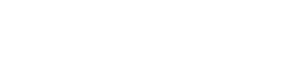# Index of dispersion (Variance to Mean Ratio)

Measures of Dispersion > Index of dispersion (Variance to Mean Ratio)

## What is the Index of dispersion?

The index of dispersion is a measure of dispersion for nominal variables and partially ordered nominal variables. It is usually defined as the ratio of the variance to the mean. As a formula, that’s:
D = σ2 / μ.

• k = number of categories in the data set (including categories with zero items),
• N = number of items in the set,
• f = number of frequencies or ratings,
• Σf2 = sum of squared frequencies/ratings.

If D = 0, all ratings fall into the same category.
IF D = 1, all ratings are equally divided between k categories.

## Example

Question: 100 people what their least favorite high school class was. Their responses were: Math(25), Economics(42), Chemistry(13), Physical Education (8), Religious Studies (13). What is the Index of Dispersion?

Step 1: Find k, the number of categories. For this example, that’s 5 (economics, chemistry, chemistry, P.E., and religious studies).

Step 2: Square the number of items in each category to get f2:

• Math (25) = 625
• Economics (42) = 1764
• Chemistry (13) = 169
• Physical Education (8) = 64
• Religious Studies (13) = 169.

Step 3: Add up all of the results in Step 2 to get Σf2:
625 + 1764 + 169 + 64 + 169 = 2791

Step 4: Insert the numbers from steps 1 – 3 into the formula and solve:Solution: A D of 0.90 indicates there is a very even spread across all categories.

## Index of Dispersion vs. Coefficient of Variation

The index of dispersion is very similar to the Coefficient of Variation, but they are not the same:

• The Coefficient of Variation is the ratio of the standard deviation to the mean. It is always dimensionless (i.e. it is a plain number without a unit) and is scale invariant (in other words, you can make changes to the length, height or other scale factors without it affecting the result).
• The Index of Dispersion is the ratio of the variance to the mean (hence the alternate name variance to mean ratio). I usually has a dimension (unless being applied to dimensionless counts) and it is not scale invariant.

References:
Walker, J. (1999). Statistics in Criminal Justice: Analysis and Interpretation. Jones & Bartlett Learning.

CITE THIS AS:
Stephanie Glen. "Index of dispersion (Variance to Mean Ratio)" From StatisticsHowTo.com: Elementary Statistics for the rest of us! https://www.statisticshowto.com/index-of-dispersion/
---------------------------------------------------------------------------Need help with a homework or test question? With Chegg Study, you can get step-by-step solutions to your questions from an expert in the field. Your first 30 minutes with a Chegg tutor is free!